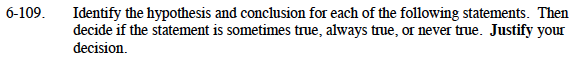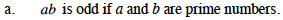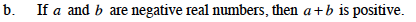### Home > CAAC > Chapter 6 > Lesson 6.3.1 > Problem6-109

6-109.Write the hypothesis and conclusion.

Hypothesis: a and b are prime numbers
Conclusion: ab is odd

Try using a few examples to test your hypothesis and conclusion.

(2)(7) = ?
(3)(11) = ?
(5)(13) = ?

Sometimes true because 2 is a prime number.Write the hypothesis and conclusion.

a and b are negative real numbers a + b is positive

Try using a few examples to test your hypothesis and conclusion.

−1 + −3 = ?
−7 + −2 = ?
−11 + −4 = ?

Never true because the set of negative real numbers is closed under addition. Closed means that if you start with 2 negatives and add them you get another negative.